The order in which you do things is often important in Maple, sometimes in rather surprising ways. One of these ways is due to the fact that the right side of an assignment statement is evaluated: the variable on the left is assigned the value that the right side evaluates to, not the right side itself. For example, consider the following sequence of statements:

> p:= 1:
q:= 1+p;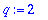A reasonable enough result: if p is 1, then q should be 2. But notice that what is assigned to q involves the value of p , not the variable itself. Maple does not remember that q was defined using p , so if p is later given a different value this will not affect q .

> p:= 3:
q;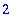Suppose, however, the order of definitions is reversed, and q is defined in terms of p while p has no assigned value. First we "unassign" p .

> p:= 'p':
q:= 1+p;Now q is defined as 1+p . When p is assigned values, the definition of q is unchanged, but when q is evaluated the result uses the current value of p .

> p:= 1:
q;> p:= 3:
q;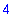Matters are complicated somewhat by arrays and procedures, which have special evaluation rules.

For arrays, see Last name evaluation . For local variables in procedures, see One level evaluation for local variables .

A procedure or function definition using proc or -> is not evaluated at the time the procedure is defined, but only when it is called. Thus global variables used in the definition are always evaluated using the current values of those variables.

> qproc:= x -> x+p:

> p:= 5:
qproc(1);If you wish to make a definition using the value of a variable at the time of definition, you can use unapply or subs . Thus each of the following procedures uses the value of p (which currently happens to be 5):

> qproc2:= unapply(x+p,x);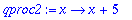> qproc3:= subs(X=p, (x -> x+X));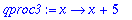> p:= 7: qproc2(1), qproc3(1);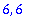Maple Advisor Database, R. Israel 1997